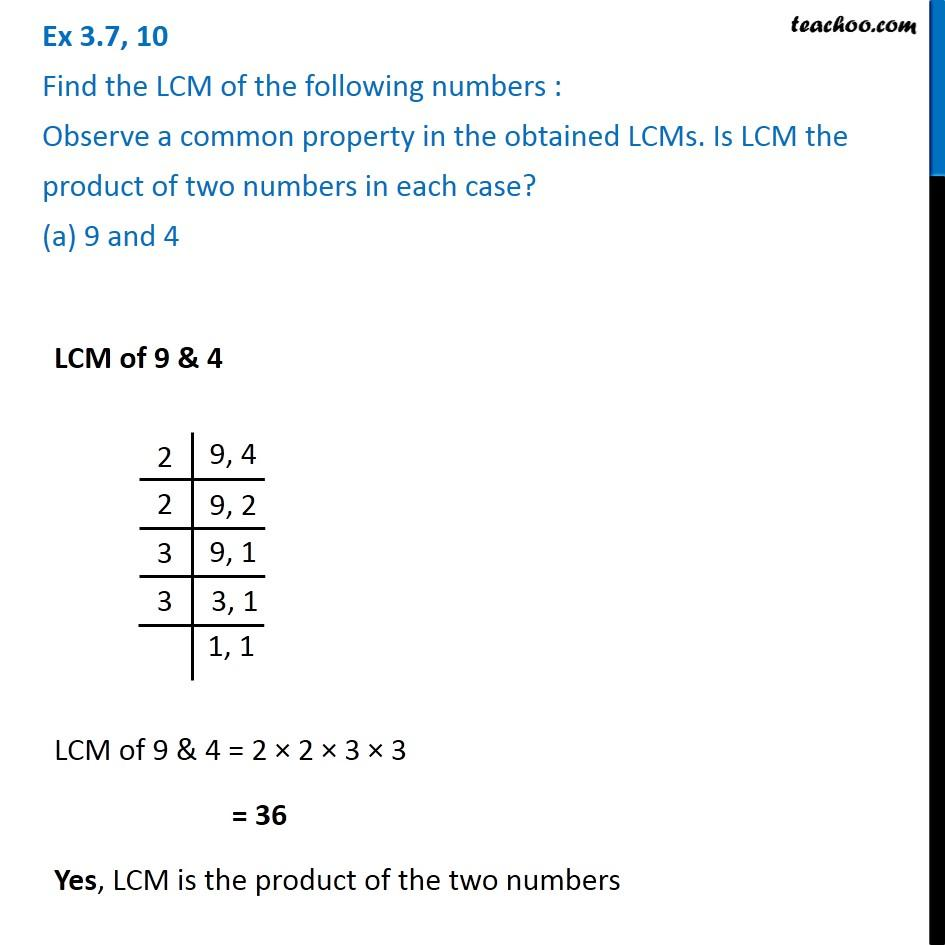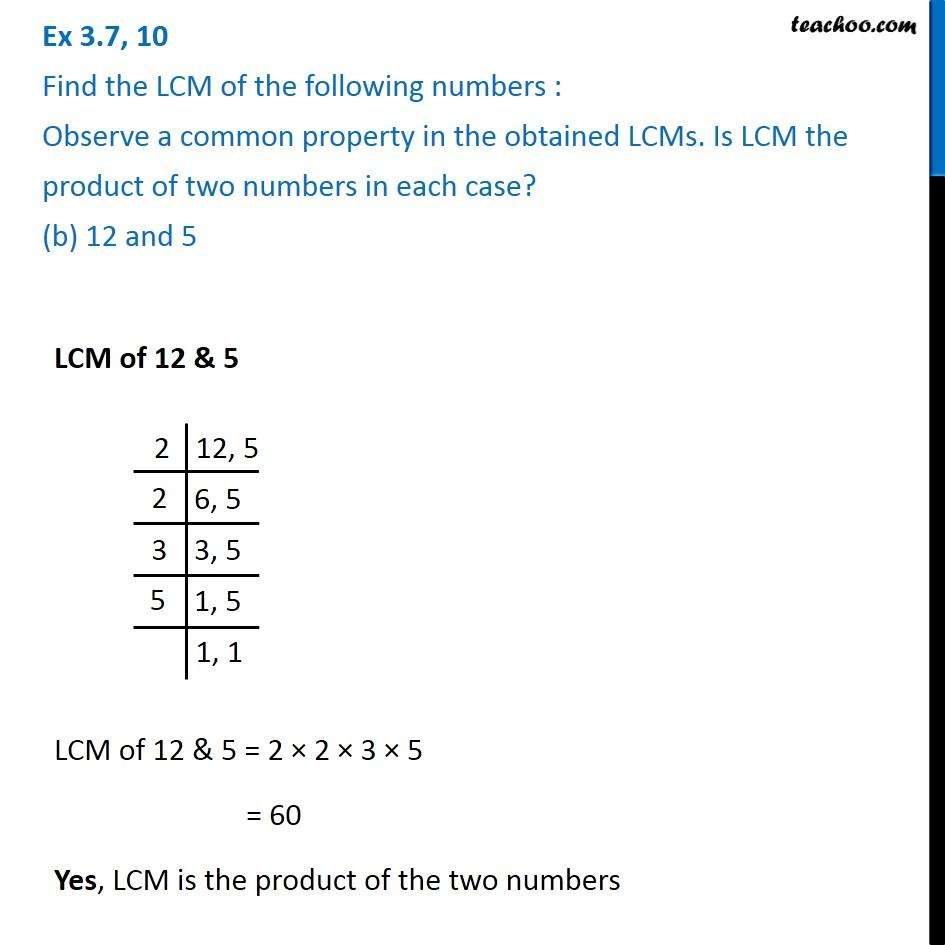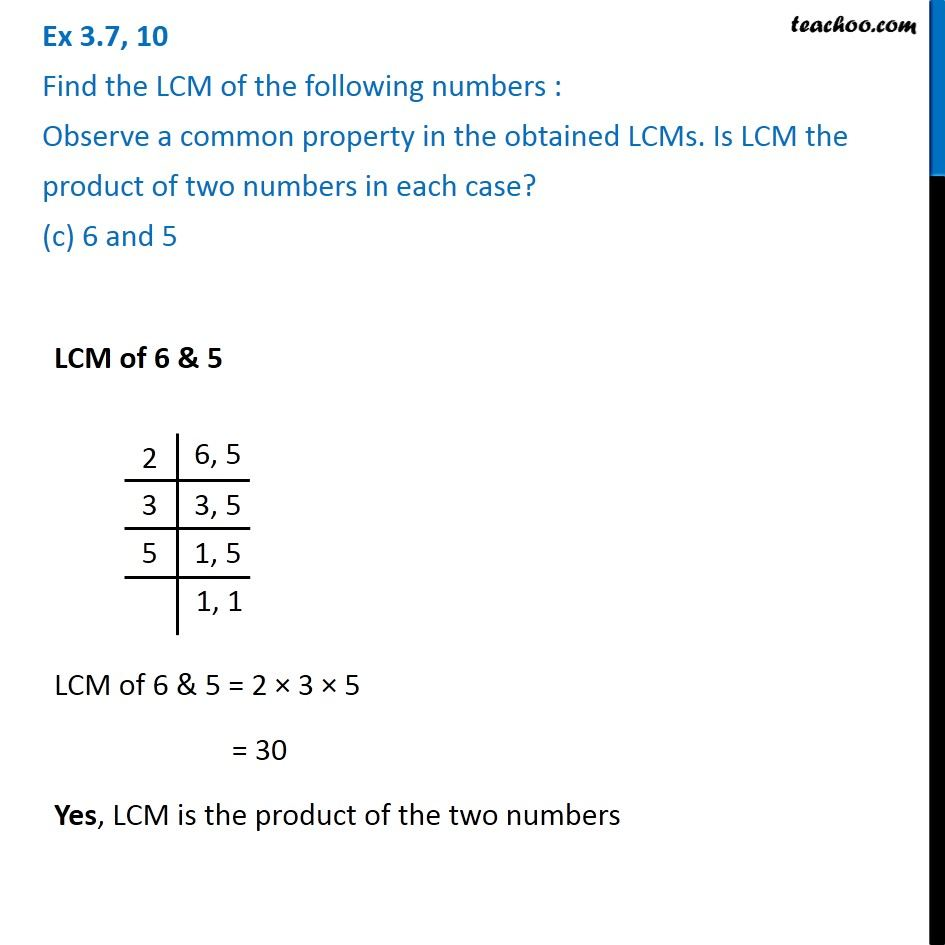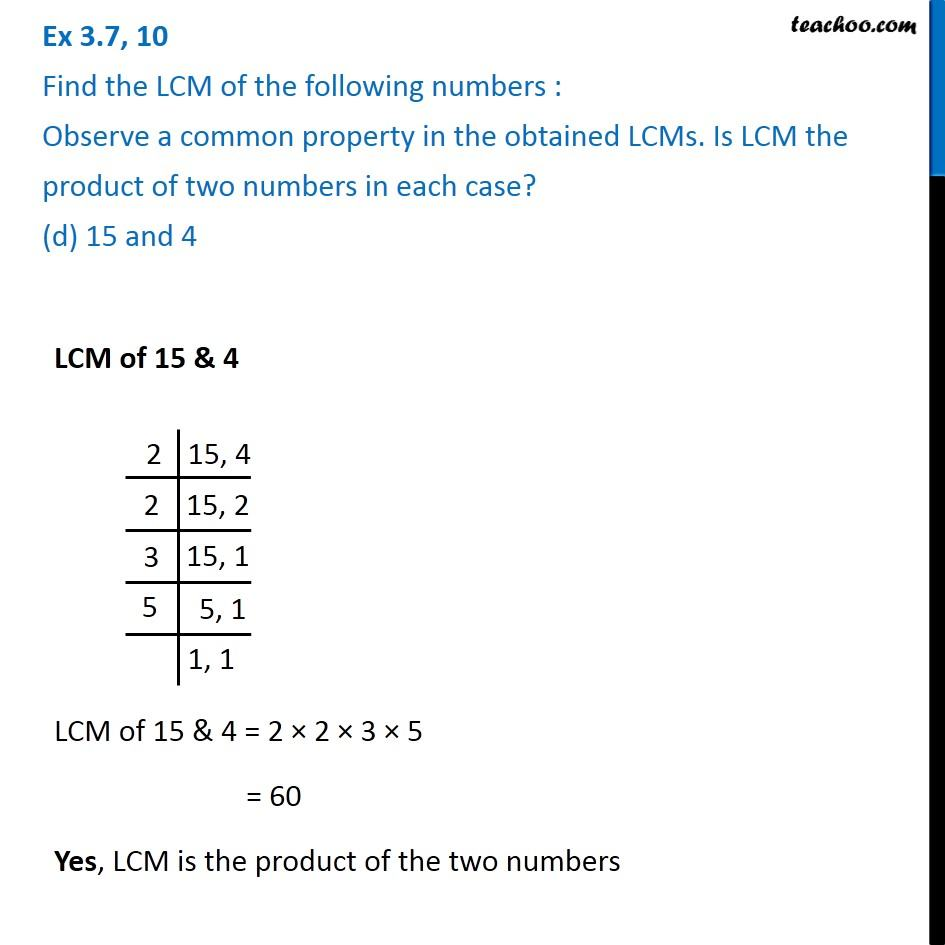Subscribe to our Youtube Channel - https://you.tube/teachoo

1. Chapter 3 Class 6 Playing with Numbers
2. Serial order wise
3. Ex 3.7

Transcript

LCM of 9 & 4 LCM of 9 & 4 = 2 × 2 × 3 × 3 = 36 Yes, LCM is the product of the two numbers LCM of 12 & 5 LCM of 12 & 5 = 2 × 2 × 3 × 5 = 60 Yes, LCM is the product of the two numbers LCM of 6 & 5 LCM of 6 & 5 = 2 × 3 × 5 = 30 Yes, LCM is the product of the two numbers LCM of 15 & 4 LCM of 15 & 4 = 2 × 2 × 3 × 5 = 60 Yes, LCM is the product of the two numbers

Ex 3.7